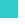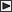BRI Research Paper

 No.64 An Evaluation Method for the Earthquake Resistant Capacity of Reinforced Concrete and Steel Reinforced Concrete Columns. M.Ozaki, Y.Ishiyama; February, 1976. 19p. Abstract An evaluation method for the earthquake resistant capacity of reinforced concrete and steel reinforced concrete columns by utilizing the force deflection relationship of column specimens subjected to axial force and repeated and reversed lateral loading of considerable intensity is proposed. An approximate response analysis for a non-linear structural system was developed based on random vibration theory and was applied to models represented by a single-degree-of-freedom system subjected to a constant white noise acceleration. The mean expected maximum response values of the models with two different natural periods 0. 1 and 0. 5 sec. were calculated. Each model has a degrading stiffness system and various hysteretic envelope slopes after four different yield point levels. The viscous damping ratio is considered to be 5% of the critical damping for the entire processes of the models, and the hysteretic damping ratio after yielding is assumed to increase according to the increase of ductility factor. The ductility factors of the models calculated by the non-linear response analysis are shown in tables and figures. The maximum strength of the linear model having the equivalent earthquake resistant capacity of a column specimen can be presumed, if the yield point,hysteretic envelope slope tangent, coefficient of hysteretic damping ratio and ductility factor of the specimen are measured by testing. A facility was designed for testing large models of reinforced concrete and steel reinforced concrete columns under action simulating gravity load and ground motion in order to standardize the testing techniques and to forestall possible errors that may be induced by the use of different types of testing facilities. An example of evaluation for the earthquake resistant capacity of a reinforced concrete column is presented by utilizing the force deflection relationship of the specimen obtained by the testing facility. It is confirmed that yield point level, hysteretic envelope slope, hysteretic damping ratio and ductility factor are the most important components of earthquake resistant capacity, when reinforced concrete or steel reinforced concrete columns are subjected to a white noise ground motion.LinksSitemapInquiryJapanese HP

BUILDING RESEARCH INSTITUTE, JAPAN